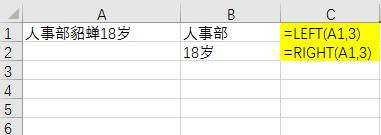# 工作中常用的Excel数字、字符处理

1、取绝对值
=ABS(A1)
A1单元格为数字

2、去空格

=TRIM(A1)

=SUBSTITUTE(A1," ","")

3、多单元格合并
=PHONETIC(B2:D2)
PHONETIC只能合并字符，不能对数字合并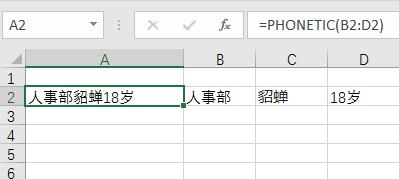4、取整数
=INT(A1)
A1单元格为数字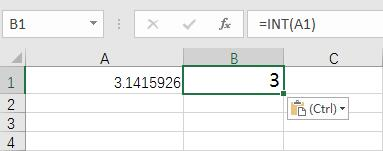5、四舍五入
=ROUND(A1,N)
A1单元格为数字；N为小数位数，譬如N=1,四舍五入1位小数；N=2，四舍五入2位小数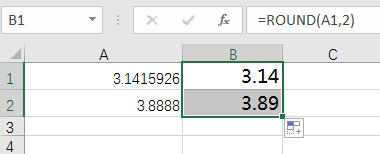6、取字符数
=LEN(A1)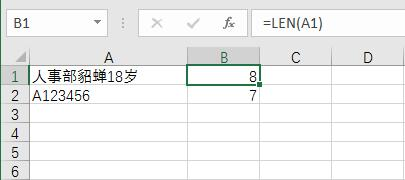7、取字符串左边或者右边的字符
=LEFT(A1,3)
=RIGHT(A1,3)